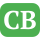CppBuzz.com

# This Code works only for small number of matrix size.

```This code is supposed to find the product of 2 matrices of which the size is entered by the user at runtime. MY code is working fine for small inputs of size of matrix say 2x2 or 3x3 if I put size more than 3 in any of the four field(row1,col1,row2,col2) it crashes. I am a beginner. And the reason I have used pointer scripting instead of subscript  is that it was the requirement for the  assignment.Also, where can I use delete command. Here is the code:
#include<iostream>
using namespace std;

int **multiply(int ** &mat1,int** &mat2,int row1,int col1,int col2,int row2)
{
int temp = 0;
//creating the resultant array
int **res_mat = new int*(&row1);  // row1 x col2 will be the size of resultant matrix
for (int i = 0; i < row1; i++)
{
*((res_mat)+i) = new int(col2);
}

for (int i = 0; i < row1; ++i)
{

for (int j = 0; j < col2; ++j)
{
*(*(res_mat + i) + j) = 0;
}
}
cout<<endl << "Calculating!!!\n"<<endl;
for (int i = 0; i < row1; ++i)
{
_beep(500, 100);
for (int j = 0; j < col2; ++j)
{
_beep(5000, 100);
for (int k = 0; k < col1; ++k)
{
_beep(1000, 100);
*(*(res_mat + i) + j) += *(*(mat1 + i) + k) * (*(*(mat2 + k) + j));
cout << res_mat[i][j]<<endl;
}
}
}
return res_mat;

}

void displayMatrix(int** mat, int row1, int col2)
{
for (int i = 0; i < row1; i++)  //i=row2
{
for (int j = 0; j < col2; j++)  //j=col2
{
cout << *(*(mat + i) + j) << '\t';

}
cout << endl;
}

}
int main()
{
int row1 = 0,
col1 = 0,
row2 = 0,
col2 = 0;

do
{
cout << "The number of columns in the first matrix must be equal to the number of rows in the second matrix.\n\n";
cout << "Rows in matrix 1: ";
cin >> row1;
cout << "Columns in matrix 1: ";
cin >> col1;
cout << endl << endl;

cout << "Rows in matrix 2: ";
cin >> row2;
cout << "Columns in matrix 2: ";
cin >> col2;
cout << endl << endl;
if (col1 != row2)
} while (col1 != row2);

int **mat1 = new int*(&row1);    // creates first matrix
for (int i = 0; i < row1; i++)
{
*((mat1) + i) = new int(col1);
}

int **mat2 = new int*(&row2); // creates second matrix
for (int i = 0; i < row2; i++)
{
*((mat2)+i) = new int(col2);
}

//*********** taking input in matrix 1************
cout << "\t\t*********** Input in matrix 1************\n\n";
for (int i = 0; i < row1; i++)  //i=row1
{
for (int j = 0; j < col1; j++)  //j=col1
{
cout << "Enter [" << i
<< "][" << j << "] : ";
cin >> *(*(mat1 + i) + j);
}
}

//*********** taking input in matrix 2************
cout << "\t\t*********** Input in matrix 2************\n\n";
for (int i = 0; i < row2; i++)  //i=row2
{
for (int j = 0; j < col2; j++)  //j=col2
{
cout << "Enter [" << i
<< "][" << j << "] : ";
cin >> *(*(mat2 + i) + j);
}
}
system("cls");
int **reciever;
reciever = multiply(mat1, mat2, row1, col1, col2, row2);

displayMatrix(reciever, row1, col2);
cout << endl << endl;

//deleting the matrices
/*for (int i = 0; i < row1; i++)
delete[](*mat1 + i);
delete[]mat1;

for (int i = 0; i < row2; i++)
delete[](*mat2 + i);
delete[]mat2;
*/

/*for (int i = 0; i < row1; i++)
delete[](*reciever + i);
delete[]reciever;*/

return 0;
}```